Queries for Gelfand-Tsetlin patterns: search statistic / browse statistics / browse maps from / browse maps to

# 1. Definition

• A Gelfand-Tsetlin pattern (or GT-pattern) is a triangular configuration of non-negative integers,

• $$\begin{array}{cccccccccccccc} x_{n1} & & x_{n2} & & \cdots & & x_{nn} \\ & \ddots & & \ddots & & & & & \\ & & x_{21} & & x_{22} \\ & & & x_{11} & \end{array}$$

with $x_{i+1,j} \geq x_{ij}$ and $x_{ij} \geq x_{i+1,j+1}$ whenever the indexing is defined.

 the 9 Gelfand-Tsetlin patterns of size (2, 4)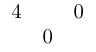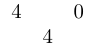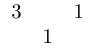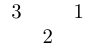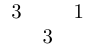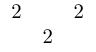[[4,0],] [[4,0],] [[4,0],] [[4,0],] [[4,0],] [[3,1],] [[3,1],] [[3,1],] [[2,2],]

• The two defining conditions ensure every row to be an integer partition and that any two adjacent rows define a skew shape.

• The shape of a GT-pattern is the partition given by its top row and its weight (or type) of the integer partition of the multiplicities of its entries. The following GT-pattern has shape $(5,4,3,1)$ and weight $(4,4,3,1,1)$:

$$\begin{matrix}5 & & 4 & & 3 & & 1 & & 0\\ & 5 & & 4 & & 3 & & 0 \\ & & 5 & & 3 & & 3 & \\ & & & 5 & & 3 \\ & & & & 4\\ \end{matrix}$$

• There is a natural bijection between GT-patterns with parameters $(l,s)$ and semi-standard Young tableaux with $s$ boxes and maximal entry at most $l$. The skew shape defined by row $j$ and $j+1$ (counting from the bottom) in a GT-pattern describes which cells in the corresponding tableau contain $j$.

# 3. Gelfand-Tsetlin polytopes

The inequalities above (with a fixed top row and fixed row sums) describe a polytope in $\mathbb{R}^{\frac{n(n+1)}{2}}$, and each GT-pattern therefore naturally corresponds to a lattice point inside this polytope. This type of polytope has integer vertices.

The usual definition of a Gelfand-Tsetlin polytope is to impose an additional restriction on the row sums. Thus, the GT-polytope $P_{\lambda,w}$ is defined as all real Gelfand-Tsetlin patterns $(x_{ij})$ such that $x_{ni}=\lambda_i$ and $\sum_i (x_{j,i}-x_{j-1,i}) = w_j$ where $x_{ij}=0$ whenever the indexing is outside the triangular array. Then, the lattice points in $P_{\lambda,w}$ are in bijection with semi-standard Young tableaux of shape $\lambda$ and type $w$, and the lattice points are counted by the Kostka numbers $K_{\lambda w}$.

The vertices of these polytopes are for small examples integral [KTT04], but there are families of GT-patterns where the GT-polytope has arbitrary large denominator, see [Lo04].

Hence, for each pair $(\lambda,w)$ the polytope can $P_{\lambda,w}$ either be

• Empty
• Integral
• Non-integral

All non-empty $P_{\lambda,w}$ contain at least one integral vertex, (this is a consequence of Knutson and Tao's proof of the Saturation conjecture). All polytopes $P_{\lambda,\lambda}$ consist of exactly one lattice point.

Since $K_{\lambda w}$ is invariant under permutation of the entries in $w$ (proved by Bender and Knuth), most research has been focused on the case when $w$ is a partition. However, $P_{\lambda,w}$ might be integral for some $w$ but non-integral for some permutation of w.

## 3.1. Tiles

In [Lo04], the notion of tiles is introduced as an important statistic on GT-patterns. The related statistic is linked to the dimension of faces of Gelfand-Tsetlin polytopes.

The tiling of a pattern is the finest partition of the entries in the pattern, such that adjacent (NW,NE,SW,SE) entries that are equal belong to the same part. These parts are called tiles, and each entry in a pattern belong to exactly one tile.

A tile is free if it do not intersect any of the first and the last row.

For example,

$$\begin{matrix}5 & & 4 & & 2 & & 1 & & 0\\ & 5 & & 3 & & 2 & & 0 \\ & & 5 & & 3 & & 2 & \\ & & & 5 & & 2 \\ & & & & 4\\ \end{matrix}$$

has 7 tiles, and the tile consisting of the two 3:s is the only free tile.

"The machinery of tilings allows us easily to find non-integral vertices of GT-polytopes by looking for a tiling with a tiling matrix satisfying certain properties. [See Lemma 2.2 in source] Then the tilings can be "filled" in a systematic way with the entries of a GT pattern that is a non-integral vertex." [Lo04]

# 4. Applications

## 4.1. Schur polynomials

The close connection between GT-patterns and Schur polynomials is quite apparent. The Schur polynomial $s_\lambda(x_1,\dotsc,x_n)$ can be expressed as a sum over lattice points in $GT(\lambda)$, the set of integral triangular GT-patterns with top row equal to $\lambda$. We have that

$$s_\lambda(x_1,\dotsc,x_n) = \sum_{G \in GT(\lambda) } x^{w(G)}$$

where $w$ is the weight of the pattern $G$.

## 4.2. Hall–Littlewood polynomials

The identity for Schur functions can be generalized to Hall–Littlewood polynomials, via a weighted version of Brion's theorem. See [FM06]

Let $\lambda$ be a partition. Then

$$P_\lambda(x;t) = \sum_{G \in GT(\lambda) } p_G(t) x^{w(G)}$$

where $p_G(t)$ is a certain statistic that depends on $G$. This theorem can also be proved via other means, see [Mac95]

## 4.3. Key polynomials

The key polynomials generalize the Schur polynomials. In [KST12], a formula for key polynomials is proved, where the sum runs over lattice points in a union of so called reduced Kogan faces of $GT(\lambda)$.

## 4.4. Schubert polynomials

It was recently proved in 1903.08275 that Schubert polynomials can be expressed as a sum over lattice points in a Minkowski-sum of GT-polytopes.

Alternatively, Schubert polynomials can also be expressed as a sum over reduced Kogan faces of a certain Gelfand-Tsetlin polytope, where each face contribute with a single monomial. The reduced Kogan faces are in bijection with pipe dreams, which are used to describe Schubert polynomials.

# 5. Remarks

It is possible to extend this definition to a parallelogram GT-pattern, in which case the GT-pattern corresponds to a skew semi-standard Young tableau. For example, here is such a GT-pattern and the corresponding skew semi-standard Young tableau: $$\begin{matrix}5 & & 4 & & 3 & & 1\\ & 4 & & 4 & & 3 & & 1\\ & & 4 & & 4 & & 2 & & 1\\ & & & 4 & & 3 & & 2 & & 1\\ & & & & 3 & & 2 & & 2 & & 0\\ & & & & & 2 & & 2 & & 0 & & 0 \\ \end{matrix} \qquad \begin{matrix} & & 1 & 2 & 5\\ & & 2 & 3\\1 & 1 & 4\\2\\ \end{matrix}.$$

The skew shape is $(5, 4, 3, 1)/(2, 2)$ and the weight is $(3, 3, 1, 1, 1)$.

## 5.1. Alternating sign matrices

There is also a correspondence between Gelfand Tsetlin patterns and Alternating Sign Matrices. In this case we use a special type of Gelfand Tsetlin pattern called a monotone triangle. Monotone triangles are Gelfand Tsetlin patterns with strict inequalities instead of weak, and the top row equals $1 2 \cdots n$.

Using the alternating sign matrix and its monotone triangle as above we have a map which gives the following Gelfand Tsetlin pattern:

\begin{align*} 1 & &2 & &3 & &4 \\ &\; \; \; \; \; \; \;1 & &\; \; \; \; \; \; \;\;3 & &\; \; \; \; \; \; \;4 & \\ & & 1 & &3 & & \\ & & &\; \; \; \; \; \; \; \;2 & & & \end{align*} which has column partial sum matrix $\begin{pmatrix}0 &1 &0 &0\\1 &0 &1 &0 \\1 &0 &1 &1 \\1 &1 &1 &1 \end{pmatrix}$ and maps to the alternating sign matrix $\begin{pmatrix}0 &1 &0 &0 \\1 &-1 &1 &0 \\0 &0 &0 &1 \\0 &1 &0 &0 \end{pmatrix}$

[FM06]   Boris Feigin and Igor Makhlin, A combinatorial formula for affine Hall–Littlewood functions via a weighted Brion theorem, Selecta Mathematica, 22(3):1703–1747, March 2016.

[KST12]   Valentina A. Kirichenko, Evgeny Yu Smirnov, and Vladlen A. Timorin, Schubert calculus and Gelfand–Zetlin polytopes, Russian Mathematical Surveys, 67(4):685, 2012.

[KTT04]   R.C. King, C. Tollu, and F. Toumazet, Stretched Littlewood-Richardson coefficients and Kostka coefficients, CRM Proceedings and Lecture Notes (2004), no. 24, 99–112..

[Lo04]   J. A. De Loera and T. B. McAllister, Vertices of Gelfand-Tsetlin polytopes, Discrete Comput. Geom. 32 (2004), no. 4, 459–470.

[Mac95]   Ian G. Macdonald, Symmetric functions and Hall polynomials, The Clarendon Press Oxford University Press, New York, second edition, 1995.

[Sta99]   R. Stanley, Enumerative Combinatorics II, Cambridge University Press (1999).

# 9. Technical information for database usage

• A GT-pattern is uniquely represented as a list of lists representing its rows from top to bottom.

• GT-patterns are parametrized by two parameters length of the first row and sum of the first row.

• The database contains all GT-pattern where the sum of the two parameters is at most 6.